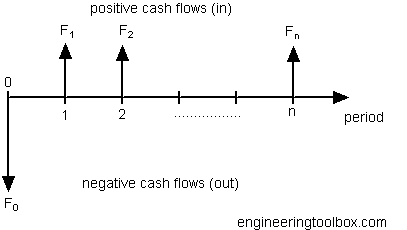Engineering ToolBox - Resources, Tools and Basic Information for Engineering and Design of Technical Applications!

# Perpetuities

## An asset providing a fixed cash flow.

A perpetuity is an asset that provides a never ending periodic fixed amount of cash flow.Endowments and trust funds and long lived assets like oil wells or gas fields can be regarded as a perpetuity.

The value of a perpetuity can be calculated as

P = F1 / (1 + i)1F2 / (1 + i)2 F3 / (1 + i)3 + ...... + Fn / (1 + i)n

= F / i                                    (1)

where

P = present value

F = F1 = F2  .. Fn  = the fixed amount of cash flow per period

i = discount rate of return (per time period)

### Example - The Value of a Company and Net Income

A company is able to deliver a net income every year of 100. Assuming that this is a perpetuity - a never ending income - the value of this cash flow (and the value of the company) with a discount rate of 10% (i = 0.10) can be calculated to

P = (100) / 0.10

= 1000

### Growing Perpetuity

If a cash flow grows in a constant rate the value of the perpetuity can be expressed as

P = F / (i - g)        (2)

where

g = growth rate of cash flow (per time period)

### Example - The Value of a Growth Company and Net Income

A growth company is able to deliver a net income first year of 200 with a net income growth rate the next years of 3% (g = 0.03). Assuming that this is a perpetuity - a never ending income - the value of this cash flow (and the value of the company) with a discount rate of 10% (i = 0.10) can be calculated to

P = (100) / (0.10 - 0.03)

= 1429

## Related Topics

• ### Economics

Engineering economics - cash flow diagrams, present value, discount rates, internal rates of return - IRR, income taxes, inflation.

## Engineering ToolBox - SketchUp Extension - Online 3D modeling!

Add standard and customized parametric components - like flange beams, lumbers, piping, stairs and more - to your Sketchup model with the Engineering ToolBox - SketchUp Extension - enabled for use with older versions of the amazing SketchUp Make and the newer "up to date" SketchUp Pro . Add the Engineering ToolBox extension to your SketchUp Make/Pro from the Extension Warehouse !

We don't collect information from our users. More about

## Citation

• The Engineering ToolBox (2009). Perpetuities. [online] Available at: https://www.engineeringtoolbox.com/perpetuity-d_1497.html [Accessed Day Month Year].

Modify the access date according your visit.

9.27.11